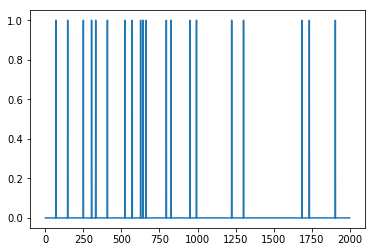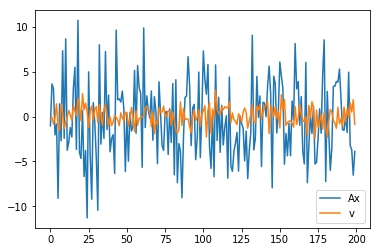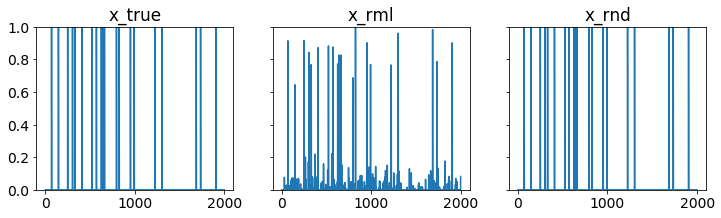# Fault detection¶

We’ll consider a problem of identifying faults that have occurred in a system based on sensor measurements of system performance.

# Problem statement¶

Each of $$n$$ possible faults occurs independently with probability $$p$$. The vector $$x \in \lbrace 0,1 \rbrace^{n}$$ encodes the fault occurrences, with $$x_i = 1$$ indicating that fault $$i$$ has occurred. System performance is measured by $$m$$ sensors. The sensor output is

$\begin{equation} y = Ax + v = \sum_{i=1}^n a_i x_i + v, \end{equation}$

where $$A \in \mathbf{R}^{m \times n}$$ is the sensing matrix with column $$a_i$$ being the fault signature of fault $$i$$, and $$v \in \mathbf{R}^m$$ is a noise vector where $$v_j$$ is Gaussian with mean 0 and variance $$\sigma^2$$.

The objective is to guess $$x$$ (which faults have occurred) given $$y$$ (sensor measurements).

We are interested in the setting where $$n > m$$, that is, when we have more possible faults than measurements. In this setting, we can expect a good recovery when the vector $$x$$ is sparse. This is the subject of compressed sensing.

# Solution approach¶

To identify the faults, one reasonable approach is to choose $$x \in \lbrace 0,1 \rbrace^{n}$$ to minimize the negative log-likelihood function

$\begin{equation} \ell(x) = \frac{1}{2 \sigma^2} \|Ax-y\|_2^2 + \log(1/p-1)\mathbf{1}^T x + c. \end{equation}$

However, this problem is nonconvex and NP-hard, due to the constraint that $$x$$ must be Boolean.

To make this problem tractable, we can relax the Boolean constraints and instead constrain $$x_i \in [0,1]$$.

The optimization problem

$\begin{split}\begin{array}{ll} \mbox{minimize} & \|Ax-y\|_2^2 + 2 \sigma^2 \log(1/p-1)\mathbf{1}^T x\\ \mbox{subject to} & 0 \leq x_i \leq 1, \quad i=1, \ldots n \end{array}\end{split}$

is convex. We’ll refer to the solution of the convex problem as the relaxed ML estimate.

By taking the relaxed ML estimate of $$x$$ and rounding the entries to the nearest of 0 or 1, we recover a Boolean estimate of the fault occurrences.

# Example¶

We’ll generate an example with $$n = 2000$$ possible faults, $$m = 200$$ measurements, and fault probability $$p = 0.01$$. We’ll choose $$\sigma^2$$ so that the signal-to-noise ratio is 5. That is,

$\begin{equation} \sqrt{\frac{\mathbf{E}\|Ax \|^2_2}{\mathbf{E} \|v\|_2^2}} = 5. \end{equation}$
import numpy as np
import matplotlib.pyplot as plt

np.random.seed(1)

n = 2000
m = 200
p = 0.01
snr = 5

sigma = np.sqrt(p*n/(snr**2))
A = np.random.randn(m,n)

x_true = (np.random.rand(n) <= p).astype(int)
v = sigma*np.random.randn(m)

y = A.dot(x_true) + v


Below, we show $$x$$, $$Ax$$ and the noise $$v$$.

plt.plot(range(n),x_true)

[<matplotlib.lines.Line2D at 0x11ae42518>]plt.plot(range(m), A.dot(x_true),range(m),v)
plt.legend(('Ax','v'))

<matplotlib.legend.Legend at 0x11aee9630># Recovery¶

We solve the relaxed maximum likelihood problem with CVXPY and then round the result to get a Boolean solution.

%%time
import cvxpy as cp
x = cp.Variable(shape=n)
tau = 2*cp.log(1/p - 1)*sigma**2
obj = cp.Minimize(cp.sum_squares(A*x - y) + tau*cp.sum(x))
const = [0 <= x, x <= 1]
cp.Problem(obj,const).solve(verbose=True)
print("final objective value: {}".format(obj.value))

# relaxed ML estimate
x_rml = np.array(x.value).flatten()

# rounded solution
x_rnd = (x_rml >= .5).astype(int)

ECOS 2.0.4 - (C) embotech GmbH, Zurich Switzerland, 2012-15. Web: www.embotech.com/ECOS

It     pcost       dcost      gap   pres   dres    k/t    mu     step   sigma     IR    |   BT
0  +7.343e+03  -3.862e+03  +5e+04  5e-01  5e-04  1e+00  1e+01    ---    ---    1  1  - |  -  -
1  +4.814e+02  -9.580e+02  +8e+03  1e-01  6e-05  2e-01  2e+00  0.8500  1e-02   1  2  2 |  0  0
2  -2.079e+02  -1.428e+03  +6e+03  1e-01  4e-05  8e-01  2e+00  0.7544  7e-01   2  2  2 |  0  0
3  -1.321e+02  -1.030e+03  +5e+03  8e-02  3e-05  7e-01  1e+00  0.3122  2e-01   2  2  2 |  0  0
4  -2.074e+02  -8.580e+02  +4e+03  6e-02  2e-05  6e-01  9e-01  0.7839  7e-01   2  2  2 |  0  0
5  -1.121e+02  -6.072e+02  +3e+03  5e-02  1e-05  5e-01  7e-01  0.3859  4e-01   2  3  3 |  0  0
6  -4.898e+01  -4.060e+02  +2e+03  3e-02  8e-06  3e-01  5e-01  0.5780  5e-01   2  2  2 |  0  0
7  +7.778e+01  -5.711e+01  +8e+02  1e-02  3e-06  1e-01  2e-01  0.9890  4e-01   2  3  2 |  0  0
8  +1.307e+02  +6.143e+01  +4e+02  6e-03  1e-06  6e-02  1e-01  0.5528  1e-01   3  3  3 |  0  0
9  +1.607e+02  +1.286e+02  +2e+02  3e-03  4e-07  3e-02  5e-02  0.8303  3e-01   3  3  3 |  0  0
10  +1.741e+02  +1.557e+02  +1e+02  2e-03  2e-07  2e-02  3e-02  0.6242  3e-01   3  3  3 |  0  0
11  +1.834e+02  +1.749e+02  +5e+01  8e-04  9e-08  8e-03  1e-02  0.8043  3e-01   3  3  3 |  0  0
12  +1.888e+02  +1.861e+02  +2e+01  3e-04  3e-08  2e-03  4e-03  0.9175  3e-01   3  3  2 |  0  0
13  +1.909e+02  +1.902e+02  +4e+00  7e-05  7e-09  6e-04  1e-03  0.8198  1e-01   3  3  3 |  0  0
14  +1.914e+02  +1.912e+02  +1e+00  2e-05  2e-09  2e-04  3e-04  0.8581  2e-01   3  2  3 |  0  0
15  +1.916e+02  +1.916e+02  +1e-01  2e-06  3e-10  2e-05  4e-05  0.9004  3e-02   3  3  3 |  0  0
16  +1.916e+02  +1.916e+02  +4e-02  7e-07  8e-11  7e-06  1e-05  0.8174  1e-01   3  3  3 |  0  0
17  +1.916e+02  +1.916e+02  +8e-03  1e-07  1e-11  1e-06  2e-06  0.8917  9e-02   3  2  2 |  0  0
18  +1.916e+02  +1.916e+02  +2e-03  4e-08  4e-12  4e-07  5e-07  0.8588  2e-01   3  3  3 |  0  0
19  +1.916e+02  +1.916e+02  +2e-04  3e-09  3e-13  3e-08  5e-08  0.9309  2e-02   3  2  2 |  0  0
20  +1.916e+02  +1.916e+02  +2e-05  4e-10  4e-14  4e-09  6e-09  0.8768  1e-02   4  2  2 |  0  0
21  +1.916e+02  +1.916e+02  +4e-06  6e-11  6e-15  6e-10  9e-10  0.9089  6e-02   4  2  2 |  0  0
22  +1.916e+02  +1.916e+02  +1e-06  2e-11  2e-15  2e-10  2e-10  0.8362  1e-01   2  1  1 |  0  0

OPTIMAL (within feastol=1.8e-11, reltol=5.1e-09, abstol=9.8e-07).
Runtime: 6.538894 seconds.

final objective value: 191.6347201927456
CPU times: user 6.51 s, sys: 291 ms, total: 6.8 s
Wall time: 7.5 s


# Evaluation¶

We define a function for computing the estimation errors, and a function for plotting $$x$$, the relaxed ML estimate, and the rounded solutions.

import matplotlib

def errors(x_true, x, threshold=.5):
'''Return estimation errors.

Return the true number of faults, the number of false positives, and the number of false negatives.
'''
n = len(x_true)
k = sum(x_true)
false_pos = sum(np.logical_and(x_true < threshold, x >= threshold))
false_neg = sum(np.logical_and(x_true >= threshold, x < threshold))
return (k, false_pos, false_neg)

def plotXs(x_true, x_rml, x_rnd, filename=None):
'''Plot true, relaxed ML, and rounded solutions.'''
matplotlib.rcParams.update({'font.size': 14})
xs = [x_true, x_rml, x_rnd]
titles = ['x_true', 'x_rml', 'x_rnd']

n = len(x_true)
k = sum(x_true)

fig, ax = plt.subplots(1, 3, sharex=True, sharey=True, figsize=(12, 3))

for i,x in enumerate(xs):
ax[i].plot(range(n), x)
ax[i].set_title(titles[i])
ax[i].set_ylim([0,1])

if filename:
fig.savefig(filename, bbox_inches='tight')

return errors(x_true, x_rml,.5)


We see that out of 20 actual faults, the rounded solution gives perfect recovery with 0 false negatives and 0 false positives.

plotXs(x_true, x_rml, x_rnd, 'fault.pdf')

(20, 0, 0)